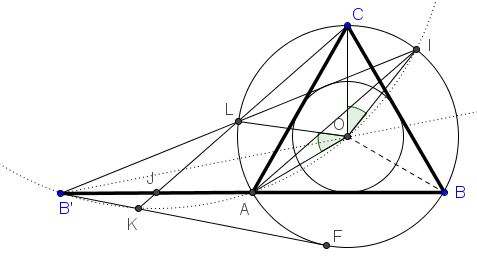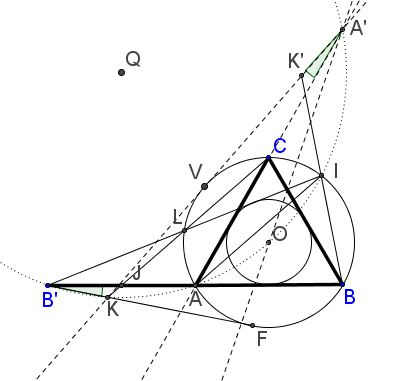# Seven Problems in Equilateral Triangle Solution to Problem 4

Given an equilateral triangle $ABC$ with the base extended to twice its length: $AB'=AB.$ Let $B'I$ (with $I$ on $(ABC))$ be the second tangent from $B'$ to the incircle of $\Delta ABC;$ $L$ the intersection of $B'I$ with $(ABC).$ $CL$ intersects $B'B$ in $J$ and $B'F$ in $K.$Prove that

1. $B'J=JA,$
2. $K$ is concyclic with $B',A,O,I.$

### Solution

Observe that, since $AB'=AB,$ due to symmetry in $B'O,$ where $O$ is the center/circumcenter of $\Delta ABC,$ $B'L=LI.$Next, let's show that $CL\parallel IA.$ Indeed, as has been shown, points $B',A,O,I$ are concyclic so that, in particular $\angle IB'A + \angle IOA = 180^{\circ}.$ On the other hand, $\angle IB'A=\frac{1}{2}(\overparen{BI}-\overparen{AL}).$ So we have

\begin{align} 180^{\circ} &= \angle IB'A + \angle IOA\\ &=\frac{1}{2}(\overparen{BI}-\overparen{AL})+(240^{\circ}-\overparen{BI})\\ &=240^{\circ}-\frac{1}{2}(\overparen{AL}+\overparen{BI})\\ &=240^{\circ}-\frac{1}{2}\overparen{AL}-\frac{1}{2}(120^{\circ}-\overparen{IC})\\ &=180^{\circ}-\frac{1}{2}\overparen{AL}+\frac{1}{2}\overparen{IC}, \end{align}

implying $\overparen{AL}=\overparen{IC}$ which makes $CL\parallel IA.$ By one of Thales' theorems and since $B'L=LI,$ $B'J=JA.$

For the next step, let's study the clockwise rotation around $O$ through $120^{\circ}.$First, by construction, $\angle B'AO=150^{\circ}$ so that $\overparen{B'AO}=360^{\circ}-2\cdot 150^{\circ}=60^{\circ}.$ It follows that $B'O$ is a side of the regular hexagon inscribed into circle $(B'AOI)$ and, as a consequence, it forms a $120^{\circ}$ angle with the adjacent side of the hexagon. In other words, the image of $B'O$ under the said rotation is a chord of the circle $(B'AOI).$ Let it be $A'O:$ $\angle B'OA'=120^{\circ}$ and $B'O=A'O.$

For convenience, let's have a notation for this rotation: $\mathcal{R}.$ So, we found that if $A'=\mathcal{R} (B')$ then $A'$ lies on $(B'AOI)$ and $\angle B'OA'=120^{\circ}$.

Further, $\mathcal{R} (AB)=CA,$ implying $\mathcal{R} (BB')=AA',$ so that $AC$ extended twice to its length ends up at $A'.$ $\mathcal{R} (B'F)=A'V,$ tangent to $(ABC)$ with $V$ on $(ABC).$ Form here, $\angle (VA',B'F)=60^{\circ}.$

$\mathcal{R} (CK)=BK',$ with $K'$ on $A'V.$ (Also, $\mathcal{R} (L)=I$ because $\angle AOC=\angle LOI$ by the first part of the proof.)

If $\overline{K}$ is the second intersection of $A'V$ with $(B'AOI)$ then $\angle AA'\overline{K}=\angle BB'F;$ this implies that $B'F$ crosses $(B'AOI)$ in $\overline{K}.$ (Otherwise, there would be two equal inscribed angles subtended by arcs of different lengths.) Both points, $K$ and $\overline{K}$ lie on $B'F,$ $\overline{K}$ is also on $(B'AOI)$. It remains to be shown that the two points coincide.

Now that we found the intersection of $A'V$ and $B'F$ to be $\overline{K},$ we can claim that $\angle A'\overline{K}F=\angle V\overline{K}F=\angle (VA',B'F)=60^{\circ},$ making $\Delta \overline{K}FV$ equilateral. And, since $\angle F\overline{K}V$ is half the difference of the two arcs $VF,$ the short one is $120^{\circ},$ from which $VF=BC:$ the two equilateral triangles are equal.

Let $Q$ be the center of $(B'AOI).$ $AI$ is the common chord of $(B'AOI)$ and $(ABC$) and, as such, is perpendicular to the line of centers $OQ.$ In addition, $L$ and $C$ are symmetric in $OQ$ as are $B'$ and $A'.$

Let $\mathcal{O}(X)$ denote the power of a point $X$ with respect to $(ABC),$ and $\mathcal{Q}(X)$ that with respect to $(B'AOI).$ Note that $\mathcal{O}(\overline{K})=(\overline{K}F)^{2}=(AB)^{2}$ and $\mathcal{Q}(\overline{K})=0.$ For $J,$ due to the first part of the proof, $\mathcal{Q}(J)=B'J\cdot AJ=(AB)^{2}/4,$ whereas $\mathcal{O}(J)=AJ\cdot BJ=3(AB)^{2}/4.$ It follows that

$\mathcal{O}(\overline{K})-\mathcal{O}(J)=\displaystyle\frac{(AB)^{2}}{4}=\mathcal{Q}(\overline{K})-\mathcal{Q}(J).$

It follows that $J\overline{K}$ is parallel to the radical axis of the two circles $(O)$ and $(Q)$ which is $AI.$ But we previously found that $AI\parallel CL,$ i.e., $AI\parallel CJ.$ Thus we conclude that $\overline{K}$ lies on exactly same straight line as $K$ and, since they both also lie on $B'F,$ they coincide.

### Acknowledgment

The proof is an expanded (probably, unnecessarily) version of the one posted by Machó Bónis at the CutTheKnotMath facebook page.